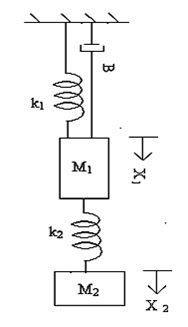• www.uptuexam.com
• GBTU Results 2014
• MTU Results 2014
• UPTU Circulars
• COP Result 2012,13,14
• UPTU Previous Papers
• GATE study material
• Robotics Projects
Thank you for visiting www.UPTUexam.com " Free download Exam materials, previous year question papers, free ebooks & Providing Important Questions To Do their Exams Well and Totally a FREE SERVICE for all engineering streams (B.Tech)."

### CONTROL SYSTEMS

CONTROL SYSTEMS :- Important questions1)      Define transfer function.
2)      What are the basic elements used for modeling mechanical rotational system?
3)      Name two types of electrical analogous for mechanical system.
4)      What is block diagram?
5)      What is the basis for framing the rules of block diagram reduction technique?
6)      What is a signal flow graph?
7)      Write Definitions /Short Notes on:
System
Control System Reference Input
Output
Feedback element
8)      Classify Control system.
9)      Differentiate between Open Loop and Closed Loop Control System.
10)  Explain importance of Feedback system.
11)  Write the rules of Block Diagram Reduction.
12)  Explain Mason’s Gain Formula.
13)  Write the differential equations governing the Mechanical system shown in fig. and determine the transfer function.14)  Determine the transfer function Y2(S)/F(S) of the system shown in fig.15)  Find the overall gain of the system whose signal flow graph is shown in fig.16) Draw a signal flow graph and evaluate the closed loop transfer function of a system
Whose block is shown in fig?17) Derive the expressions and draw the response of first order system for unit step input.
18) Draw the response of second order system for critically damped case and when input is unit step.
19)  Derive the expressions for Rise time, Peak time, and Peak overshoot. A potential control system with velocity feedback is shown in fig. What is the response of the system for unit step input?20) For a unity feedback control system the open loop transfer function
G(S) = 10(S+2)/ S2 (S+1). Find
(a) Position, velocity and acceleration error constants.
(b) The steady state error when the input is R(S) where R(S) =3/s –2/s2+1/3s3
21) What is frequency response?
22) What are advantages of frequency response analysis?
23) What are frequency domain specifications?
24) Define Resonant Peak.
25) What is resonant frequency?
26) Define Bandwidth.
27) What is cut-off rate?
28) Define gain margin.
29) Define phase margin.
30) What is phase and Gain cross-over frequency?
31) What is Bode plot?
32) Define corner frequency.
33) What are the advantages of Bode Plot?
34) Sketch the Bode plot and hence find Gain cross over frequency, Phase cross over frequency, Gain margin and Phase margin.
G(S) = 0.75(1+0.2S)/ S (1+0.5S) (1+0.1S)
35) Sketch the polar plot for the following transfer function and find Gain cross over frequency, Phase cross over frequency, Gain margin and Phase margin.
G(S) = 400/ S (S+2) (S+10)
36) What is the necessary and sufficient condition for stability?
37) What is routh stability condition?
38) What is Nyquist stability criterion?
39) Using Routh criterion determine the stability of the system whose characteristics
equation is s4+8s3+18s2+16s+5 =0 .
40) Construct Nyquist plot for a feedback control system whose open loop transfer function is given by G(S)H(S) =5/ S(1-S).comment on the stability of open loop and
closed loop transfer function.
41) Sketch the Nyquist plot for a system with the open loop transfer function
G(S)H(S) =K(1+0.5S) (1+S) / (1+10S) (S-1).determine the range of values of K for which the system is stable.
42) What are state variables?
43)  What is the state space?
44) What are phase variables?
45) What is a state vector?
46) Test the controllability & observability of the system whose state space representation is given as,47) Determine the state variable representation of the system whose transfer function is
given as Y(s) / U(s) = 2s2+8s+7 / (s+2) 2 (s+1)